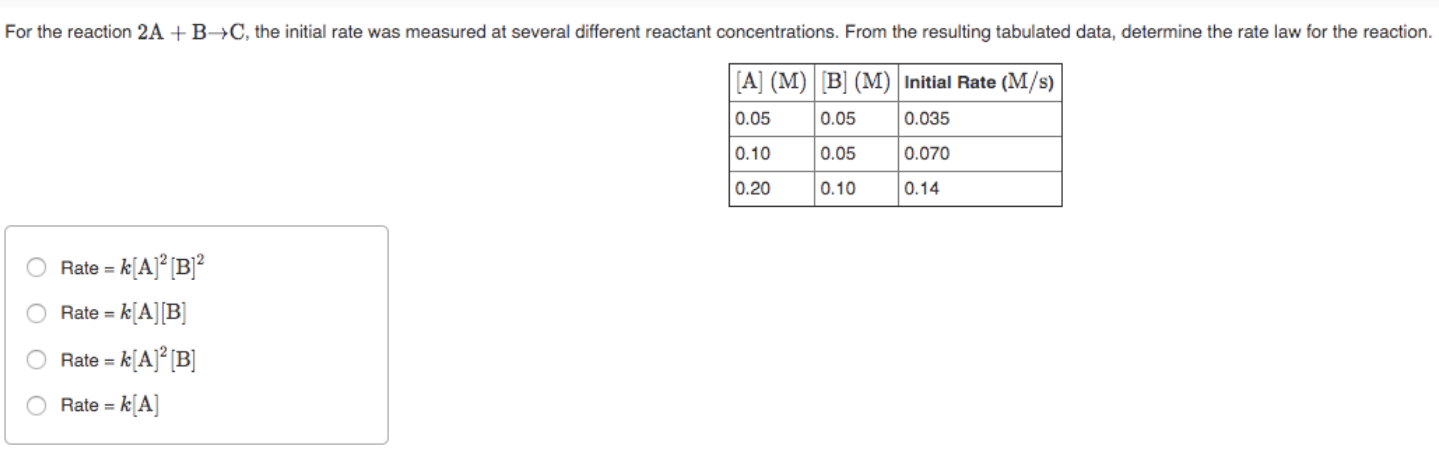# For the reaction 2A + B→C, the initial rate was measured at several different reactant concentrations. From the resulting tabulated data, determine the rate law for the reaction. a) Rate = k[A]^2[B]^2 b)Rate = k[A][B] c) Rate = k[A]^2[B] d)Rate = k[A]